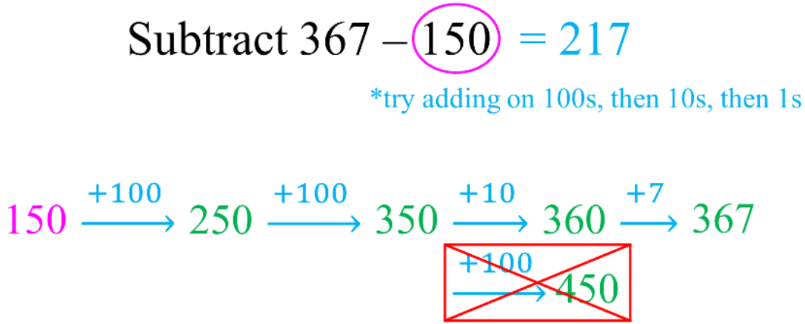# Subtraction strategies#### Everything You Need in One Place

Homework problems? Exam preparation? Trying to grasp a concept or just brushing up the basics? Our extensive help & practice library have got you covered.#### Learn and Practice With Ease

Our proven video lessons ease you through problems quickly, and you get tonnes of friendly practice on questions that trip students up on tests and finals.#### Instant and Unlimited Help

Our personalized learning platform enables you to instantly find the exact walkthrough to your specific type of question. Activate unlimited help now!

0/4
##### Intros
###### Lessons
1. Introduction to Subtraction Strategies:
2. Mental math and differences of 10
3. Subtraction strategy #1: friendly number with adjustment
4. Subtraction strategy #2: friendly number by adding to both numbers
5. Subtraction strategy #3: counting on from the first number
0/19
##### Examples
###### Lessons
1. Subtracting by breaking up the subtrahend
Subtract by breaking up the number being taken away.
Fill in the blanks by following the example: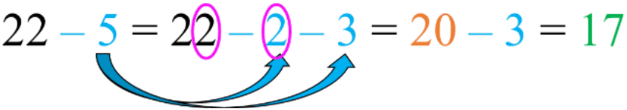1. 57 - 9 = 57 - 7 - ____ = 50 - ____ = ____
2. 64 - 7 = 64 - ____ - ____ = 60 - ____ = ____
3. 38 - 9 = 38 - ____ - ____ = 30 - ____ = ____
4. 92 - 8 = 92 - ____ - ____ = ____ - _____ = _____
2. Subtracting by 9 strategy
Subtract 9 by: subtracting 10 first, then putting 1 back
1. 17 - 9 = ____
17 - 10 = ____ + 1 = ____
2. 42 - 9 = ____
42 - 10 = ____ + 1 = ____
3. 35 - 9 = ____
35 - 10 = ____ + 1 = ____
3. Subtracting by 8 strategy
Subtract 8 by: subtracting 10 first, then putting 2 back
1. 15 - 8 = ____
15 - 10 = ____ + 2 = ____
2. 63 - 8 = ____
63 - 10 = ____ + 2 = ____
3. 47 - 8 = ____
47 - 10 = ____ + 2 = ____
4. Subtracting using friendly numbers with adjustment
Subtract by changing one number into a "friendly" number and adjust. Fill in the blanks.
1.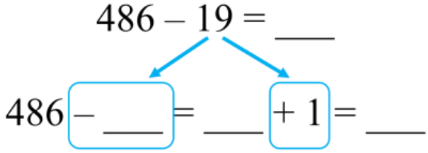2.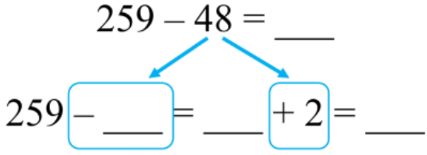3.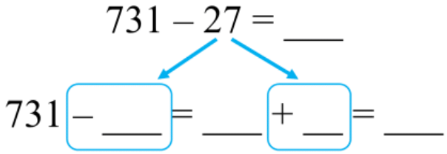5. Subtraction strategy: make friendly numbers by adding to both numbers
Subtract: make "friendly" numbers by adding to both numbers. Fill in the blanks.
1.2.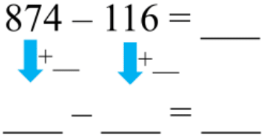3.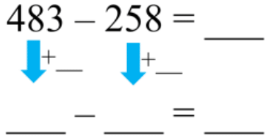6. Subtraction strategy: counting on from the second number
Subtract by counting on from the second number. Show your work.
1. 573 - 252 = ____
2. 408 - 126 = ____
3. 847 - 639 = ____
0%
##### Practice
###### Free to Join!
StudyPug is a learning help platform covering math and science from grade 4 all the way to second year university. Our video tutorials, unlimited practice problems, and step-by-step explanations provide you or your child with all the help you need to master concepts. On top of that, it's fun - with achievements, customizable avatars, and awards to keep you motivated.
• #### Easily See Your ProgressWe track the progress you've made on a topic so you know what you've done. From the course view you can easily see what topics have what and the progress you've made on them. Fill the rings to completely master that section or mouse over the icon to see more details.
• #### Make Use of Our Learning Aids###### Practice Accuracy

Get quick access to the topic you're currently learning.

See how well your practice sessions are going over time.

Stay on track with our daily recommendations.

• #### Earn Achievements as You LearnMake the most of your time as you use StudyPug to help you achieve your goals. Earn fun little badges the more you watch, practice, and use our service.
• #### Create and Customize Your AvatarPlay with our fun little avatar builder to create and customize your own avatar on StudyPug. Choose your face, eye colour, hair colour and style, and background. Unlock more options the more you use StudyPug.
###### Topic Notes

In this lesson, we will learn:

• The subtraction strategy of: (1) using a friendly number with adjustment, (2) adding to both numbers to create a friendly number, and (3) counting on from the first number (subtrahend)
• Understanding subtracting by 9

Notes:

• Method 1: change into a "friendly" number then adjust (by adding)
• Round one the number being taken away to the nearest multiple of 10
• Then adjust by adding how much you rounded by to get the answer• Method 2: make "friendly" numbers by adding to both numbers
• Add to one of the numbers so that it becomes the nearest multiple of 10
• Add the same amount to the other number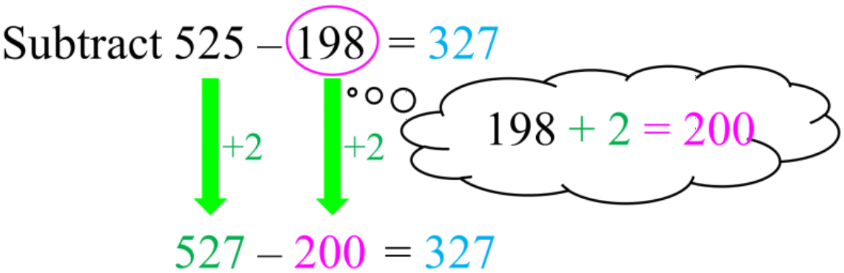• Method 3: subtracting by counting on
• Start with the number you're taking away (the second number)
• Then count up until you get the first number